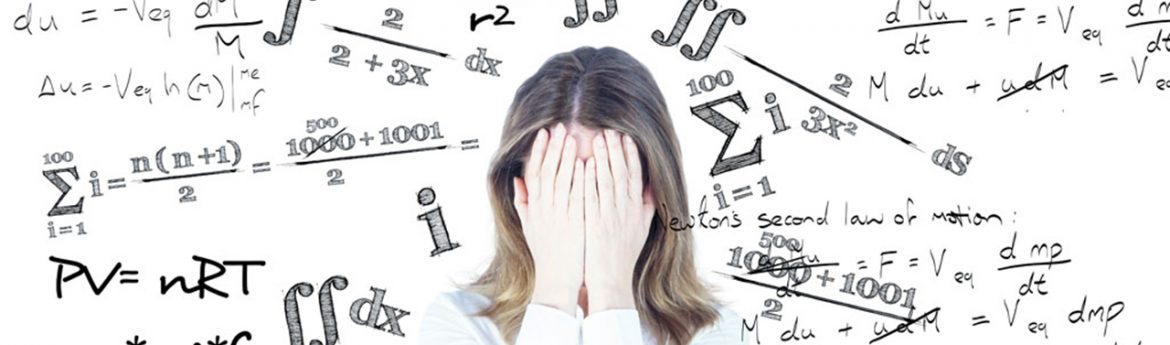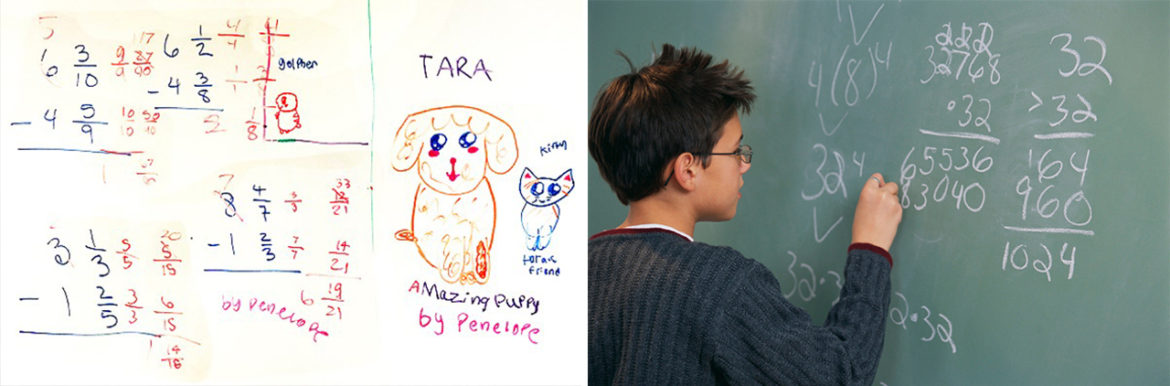Many parents fear that their child has math anxiety and call with this scenario: My child does all of her homework and has an “A” for her homework grade. Yet she receives C’s, D’s or F’s on her exams. Do you think she has test or math anxiety?

The answer to this question, in my experience, varies. Since homework is graded on completion, an “A” grade means the homework is done and does not measure how well the student understands it. A parent does not receive feedback on how well their child is understanding the math until a quiz or test is given over that material. To help your child learn and make sure he or she is understanding their homework, have them do all of the odd problems, since these usually have answers in the back of the book. Have the student check their answers and if they are making mistakes, have them keep practicing until they are able to do the problems correctly. This may mean finding additional problems to practice with outside of their text book. When first learning the material, have your child do the examples in the book. They can first follow the examples closely and then do them on their own. Many students think by reading an example or “understanding” what a teacher said, they understand the math. This is called passive understanding and does not necessarily mean they understand the math well enough to solve problems on a test over the material. Also, many times students do problems in groups in class. Students can copy each other and not understand how to do the problems on their own.

Another reason a student may score poorly on a math test is due to gaps in their math knowledge in math from prior classes. This is very common. In fact there are whole areas of research on areas called “math misconceptions,” areas of math that most students are confused about, even after they have been taught how to do the topic correctly. I find that even if a child understands their current homework and math lessons, they may do poorly on an exam due to mistakes from gaps in prior knowledge. There are a number of topics in math--order of operations, negative numbers, fractions and the like--that many students, even calculus students, have difficulty with.

A third reason may be that the child actually has test anxiety! We can discuss this in person.

READ MORE ABOUT: Does Our Approach To Teaching Math Fail Even The Smartest Kids?